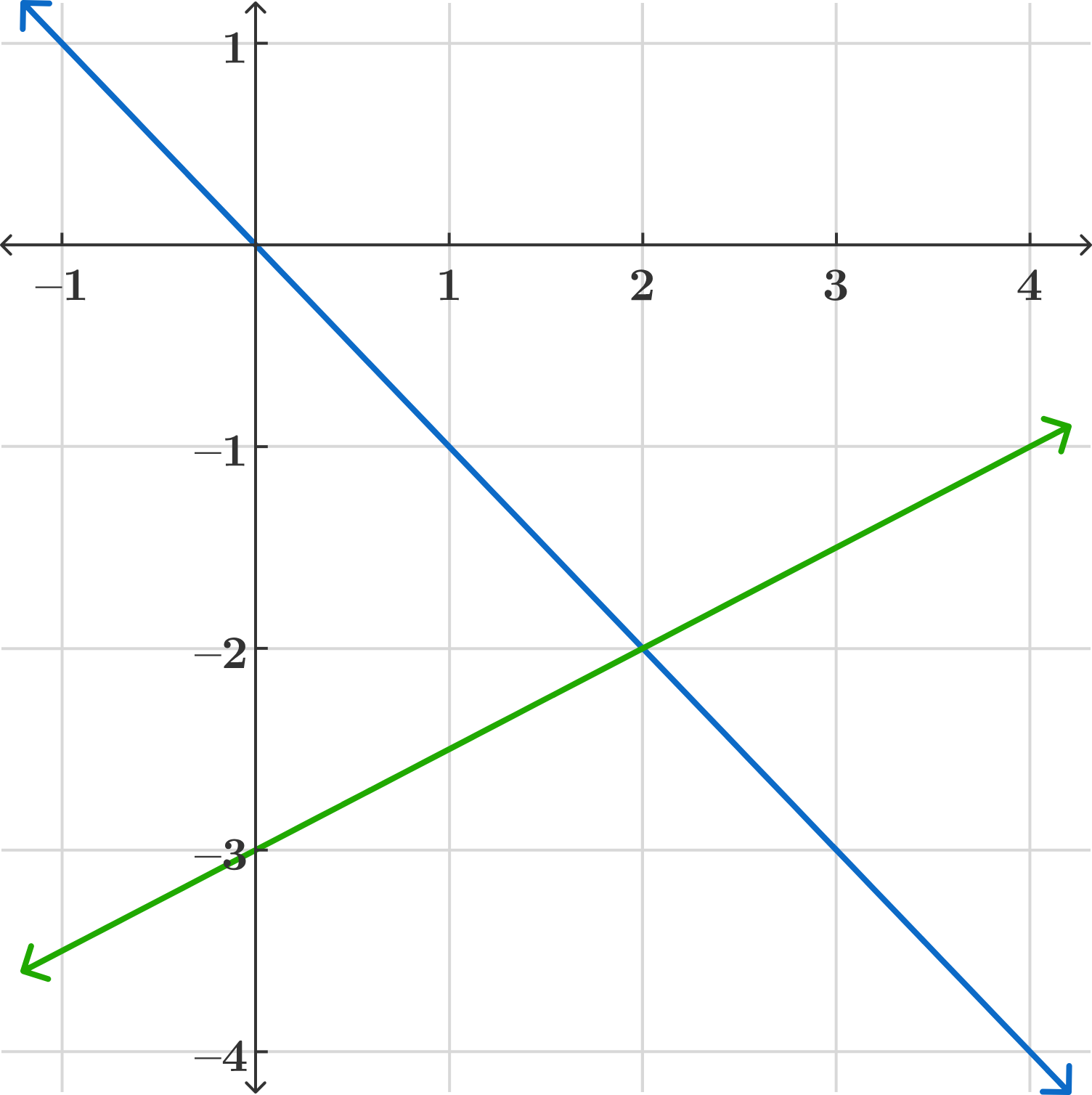### Introduction to Linear Algebra

To begin our study of linear algebra, we’ll look at a simple case: a system of two linear equations with two unknowns. Though solving this system is quite easy, there are still quite a few interesting concepts and ideas that come up when we think deeply about the process. These concepts and ideas play a larger role when we look at more general systems of linear equations later.

So let’s start with the system \begin{aligned} x-2y &= 6 \\ 3x+y &= 4. \end{aligned} The first idea we might have is to solve for one of the variables. Let’s isolate $x$ in the first equation and use it to solve $y$ in the second. After isolating $x$ in the first equation we get $x = 6+2y.$ Now we can plug that into the second equation and solve: \begin{aligned} 3(6+2y)+y &= 4 \\ 18+7y &= 4 \\ y &= -2. \end{aligned} Solving for $x$ gives $x=2,$ so we have obtained a unique solution, $x=2,y=-2.$

The next few problems are going to look at this exercise in several different ways: finding a different way to solve it, interpreting it geometrically, and imagining what might happen if we tweak some of the numbers.

# Two Linear Equations in Two Unknowns

Another standard way to do the problem is similar, in that its goal is to eliminate a variable, but it achieves this goal slightly differently. That is, we can multiply both sides of one of the equations by a constant, and then add or subtract the two equations.

\begin{aligned} x-2y &= 6 \\ 3x+y &= 4. \end{aligned}

For instance, if we want to eliminate $x,$ we can take $3$ times the first equation minus the second equation: \begin{aligned} 3x-6y &= 18 \\ 3x+y &= 4 \end{aligned} so \begin{aligned} (3x-6y)-(3x+y) &= 18-4 \\ -7y &= 14 \\ y &= -2 \end{aligned} as before. (Also, substituting $y = -2$ back into one of the original equations and solving gets $x = 2 .)$

Just to make sure we’re paying attention, let’s say we wanted to eliminate $y$ instead. Which combination of equations shown below would do that?

\begin{aligned} \text{Equation 1: }x-2y &= 6 \\ \text{Equation 2: }3x+y &= 4. \end{aligned}

# Two Linear Equations in Two Unknowns

Which tweaks to the original system of equations would change the number of solutions?

Remember, we started with \begin{aligned} x-2y &= 6 \\ 3x+y &= 4. \end{aligned} Let’s change the number “$3$” in the second equation to something else, and see what effect that has on the number of solutions. Remember that our original system has exactly one solution, $x=2,y=-2.$

What number should we change the “$3$” to in order to create a system of equations that doesn’t have exactly one solution?

# Two Linear Equations in Two Unknowns

We’ll come back to algebra shortly, but let’s pause to think about what a pair of linear equations mean geometrically.

Each of the two equations involving $x$ and $y$ cuts out a line in the coordinate plane. For example, consider the system $x+y = 0 \ , \ y - \frac{1}{2} x = - 3.$\begin{aligned} y &= -x \\ y &= \frac{1}{2}x-3 \end{aligned}

The solution to this system (like the original) is the intersection of these two lines, which is the point $(2,-2).$

Now think about all of the different ways that any two lines can intersect. Use that to answer the following question:

Suppose we have a system of two linear equations with real number coefficients in two real unknowns. Which one of the following options is impossible?

# Two Linear Equations in Two Unknowns

Now that we have some geometric intuition, let’s try to generalize. Say we have a system of two equations of the form \begin{aligned} 2x+by &= m \\ 3x+dy &= n. \end{aligned} What condition guarantees that the system will have exactly one solution?

Assume that it has at least one solution.

# Two Linear Equations in Two Unknowns

If we are even more general, we can consider the system of equations \begin{aligned} ax+by &=m \\ cx+dy &= n. \end{aligned}

Expressed in slope-intercept form:

\begin{aligned} y&=-\frac{a}{b}x+\frac{m}{b} \\\\ y&=-\frac{c}{d}x+\frac{n}{d} \end{aligned}

A system of two linear equations has a unique solution if slopes are different:

$\frac{a}{b}\neq \frac{c}{d} \implies ad-bc\neq 0.$

The system has no solutions if slopes are equal $(ad-bc=0 ),$ but the $y$-intercepts are different: $\frac{m}{b}\neq\frac{n}{d} \implies dm-bn\neq 0$

The system has infinite solutions if slopes are equal $(ad-bc=0 ),$ and $y$-intercepts are equal $(dm-bn=0).$

So there are at least two important takeaways: one is the useful fact that the “degeneration” of the system of equations can be boiled down to looking at a certain algebraic expression in the coefficients, $ad-bc$ in this case, and deciding whether it is nonzero. This turns out to generalize to larger systems of equations.

The second takeaway is our intuition about the behavior of these systems. Because a “randomly” chosen real number is almost always nonzero, we should expect that a “random” system of two equations in two unknowns will have a unique solution. (Geometrically speaking, two “random” lines will almost always not be parallel, and hence will intersect in exactly one point.) This intuition will also follow us as we explore larger systems of equations.

×